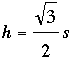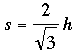SEARCH HOMEMath Central Quandaries & QueriesQuestion from Michelle, a parent: My daughter and I are having trouble with this problem...All it says i solve thr formula for the indicated variable. Height of an Equilateral Triangle. Solve for s: H= square root of 3/2 * SMichelle,

If you have an equilateral triangle with each side of length s units then you can find the height, h, of the triangle using the expressionThe instruction "Solve for s" is asking you to manipulate this equation to arrive at the form

s = some expression involving h.

To do this I would first take the original expressionand multiple both sides by 2 to obtain

2h = √3 s.

Now divide both sides by √3 and the expression becomes

(2h)/√3 = s,

or written more neatlyI hope this helps,
PennyMath Central is supported by the University of Regina and The Pacific Institute for the Mathematical Sciences.